Next: 1.4 Nanostructured Materials for Thermoelectrics Up: 1. Introduction Previous: 1.2 Device Efficiency   Contents

# 1.3 Thermoelectric Materials

Bismuth telluride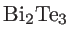, a narrow bandgap semiconductor, is one of the most commonly used thermoelectric materials . It has been shown that by adding antimony telluride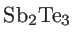and bismuth selenide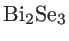to, it is possible to obtain a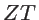of around unity. In turn, it was shown that by alloying ofwith bismuth selenide and antimony telluride, the thermal conductivity decreases, without significant degradation of the electrical conductivity . As mentioned in Sec. 1.2, working at high temperature is advantageous for energy conversion efficiency. However, the alloys of bismuth telluride are not suitable at temperature higher than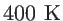. Common thermoelectric materials for higher temperatures are lead telluride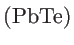and silicon-germanium alloys. These materials havebetween 0.5 and 1.1, depending on the temperature and the type of material (whether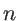-type or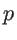-type) . As a result, the average efficiency of current thermoelectric generators is about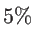.

Good thermoelectric materials should simultaneously have a high Seebeck coefficient, a high electrical conductivity, and a low thermal conductivity. While each property ofcan individually be changed by several orders of magnitude, the interdependence and coupling between these properties in bulk materials have made it extremely difficult to increase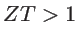. In the case of bulk materials, assuming the effective mass approximation and Fermi-Dirac statistics, one can relate the Seebeck coefficient and the electrical conductivity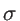to the carrier concentrationas :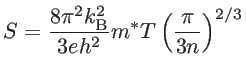(1.14)

and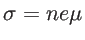(1.15)

where,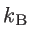is the Boltzmann constant,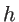the Planck constant,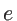the elementary charge,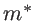the effective mass, and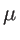the carrier mobility. In addition, the Wiedemann-Franz law relates the thermal conductivity of charge carriers to the electrical conductivity by: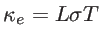(1.16)

whereis the Lorenz number: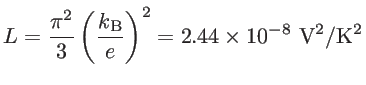(1.17)

The electrical conductivity is proportional to the carrier concentration, whereas the Seebeck coefficient is inversely proportional to the carrier concentration. Therefore, if one tries to increaseby increasingand thus, one may lose the gain through the reduction of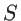(and increase in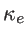). Figure 1.6 schematically shows that insulators have high Seebeck coefficient and extremely low electrical conductivity, whereas metals have high electrical conductivity and a low Seebeck coefficient . Therefore, in semiconducting materials a finite maximum of the thermoelectric power factor is achieved. As a result, the most effective way to enhance the thermoelectric figure of merit of bulk materials is to decrease the lattice contribution to the thermal conductivity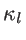. However, thermal conductivity reduction, without decreasing the power factor, was not possible for a long time (in all efforts up to the 1990s), and thevalues were limited to unity. This translates to low conversion efficiencies and limited applications for thermoelectricity.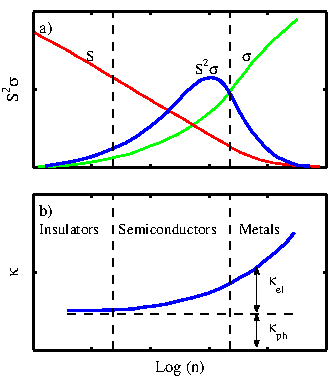Next: 1.4 Nanostructured Materials for Thermoelectrics Up: 1. Introduction Previous: 1.2 Device Efficiency   Contents
H. Karamitaheri: Thermal and Thermoelectric Properties of Nanostructures